This post is about chordal graph and semidefinite programs (SDP), one of the research topic I found very interesting. The purpose of this post is to help people understand the basic concept of chordal graph and how is chordal graph useful in solving semidefinite programs. This post is divided into three main topics:

Given a standard form semidefinite program (SDP) and its dual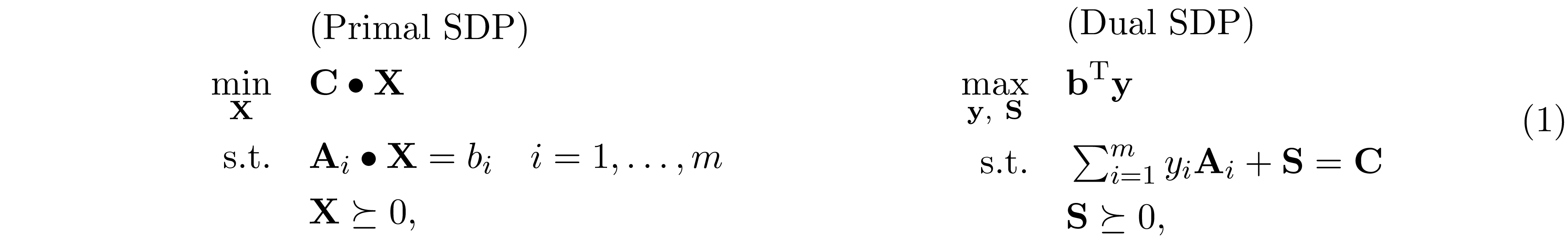where ${\bf A}_i,\ {\bf C},\ {\bf X},\ {\bf S}\in\mathrm{S^n}$, ${\bf b},\ {\bf y}\in\mathbb R^n$ and ${\bf C}\bullet{\bf X}=\mathrm{Tr}({\bf C}{\bf X})$. The fundamental difficulty of solving SDP is that the variable ${\bf X}$ is generally dense, even in the case when ${\bf C}$ and ${\bf A}_i$'s are sparse. It might seem straightforward to exploit the sparsity pattern in the dual problem, where the slack variable ${\bf S}$ has the (aggregated) sparsity pattern of ${\bf C},\ {\bf A}_1,\ldots ,{\bf A}_m$. Nonetheless, compute the gradient of log-barrier function $\phi({\bf S})=\log\det{{\bf S}}$ requires the inverse of ${\bf S}$, which is generally a dense matrix.

A sparsity pattern $\mathrm{E}=\{(i,j)\}$ is a set of position, and a matrix ${\bf A}$ is said to have the sparsity pattern of $\mathrm{V}$ if for all $(i,j)\notin\mathrm{E}$ we have $A_{i,j}=0$. It is assume that all the diagonal entires are in $\mathrm{E}$.

Although SDP can be efficiently solved by interior-point method (IPM) in just a few iterations, however, at each iteration of IPM, we have to solve a normal equationto evaluate the Newton step $\Delta{\bf y}$. In general, ${\bf H}\in\mathrm{S^m}$ is fully dense; therefore $O(m^3)$ time is required to form ${\bf H}$ and solve $\Delta{\bf y}$, this result in $O(n^6)$ time complexity per-iteration because $n\leq m^2$ and $O(n^{6.5}\log(1/\epsilon))$ time to obtain the $\epsilon$-accuracy solution using IPM.

When the SDP is chordal sparse. That is, the (aggregated) sparsity pattern of ${\bf C},{\bf A}_1,\ldots,{\bf A}_m$ is chordal sparse. We can achieve lower computational complexity to form ${\bf H}$ and solve $\Delta{\bf y}$ at each iteration of IPM. In this blog post, we elaborate a method to exploit chordal structure in SDP proposed by Zhang et al. 1.

Notation: Uppercase (lowercase) bold face letters indicate matrices (column vectors). $A_{ij}$ denotes the $(i,j)$-th element of ${\bf A}$. ${\rm Tr}(\cdot)$ denotes the trace of the matrix. $\mathrm{G(V,E)}$ denotes a graph with vertex set $\mathrm V$ and edge set $\mathrm E\subseteq \mathrm{V\times V}$. $\mathrm{S}^{n}$ is the set of symmetric matrices of order $n$. $\mathrm{S_+^n}$ and $\mathrm{S_{++}^n}$ are the sets of positive semidefinite, positive definite matrices, respectively. $\mathrm{S_E^n}$ is a set of symmetric matrices that have sparsity pattern $\mathrm E$. $\mathrm{S_{E,+}^{n}}$ and $\mathrm{S_{E,++}^{n}}$ are the sets of positive semidefinite and positive definite matrices in $\mathrm{S_E^n}$.

In many applications, we can break the underlying problems into solving a series of sparse normal equationswhere ${\bf A}$ is sparse and ${\bf A}\succ 0$. A standard approach to solve ${\bf x}$ is to perform Cholesky factorization on ${\bf A}={\bf L}{\bf L}^\mathrm{T}$, and then solve ${\bf L}{\bf d}={\bf b}$ following by ${\bf L}^\mathrm{T}{\bf x}={\bf d}$. Since ${\bf L}$ is a lower triangular matrix, solving ${\bf L}{\bf d}={\bf b}$ and ${\bf L}^\mathrm{T}{\bf x}={\bf d}$ only requires back substitution, the computational complexity to solve ${\bf x}$ is cubic time if ${\bf L}$ is dense, but achieve linear time if ${\bf L}$ is sparse. In this section, we will show that if ${\bf A}$ has chordal structure, then its sparsity structure remains the same after Cholesky factorization.

An undirected graph is chordal if every induce cycle has length 3.

To understand chordal structure, one must learn the concept of vertex elimination. Given an undirected graph $\mathrm{G(V,E)}$, the vertex elimination is defined as follow.

1. Pick any vertex $v\in\mathrm V$ and remove it from the graph.
2. Connect all the neighbors of $v$ together.
3. Repeat step 1 and 2 until $\mathrm V=\{\emptyset\}$.

The number of edges introduced during the process of vertex elimination depends on the order of elimination.

Consider a star graphCase 1: elimination order $=(v_1\to v_2\to v_3\to v_4\to v_5)$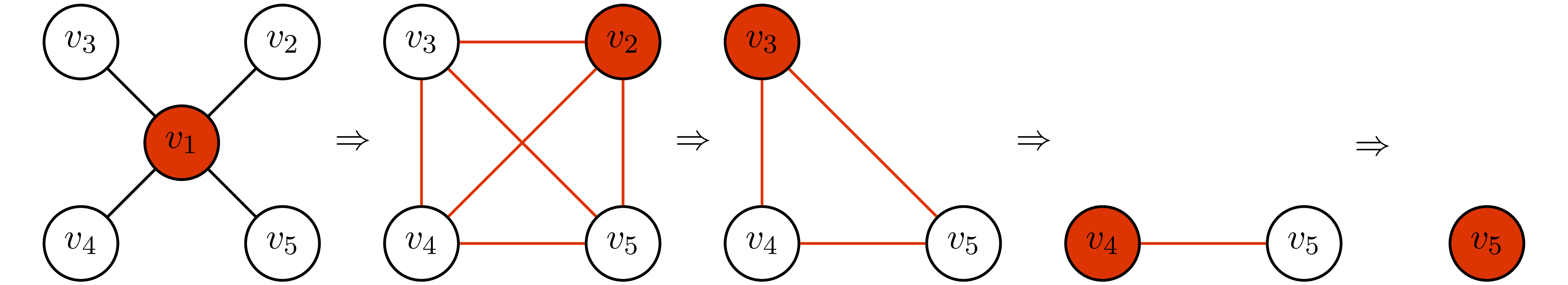Case 2: elimination order $=(v_2\to v_3\to v_4\to v_5\to v_1)$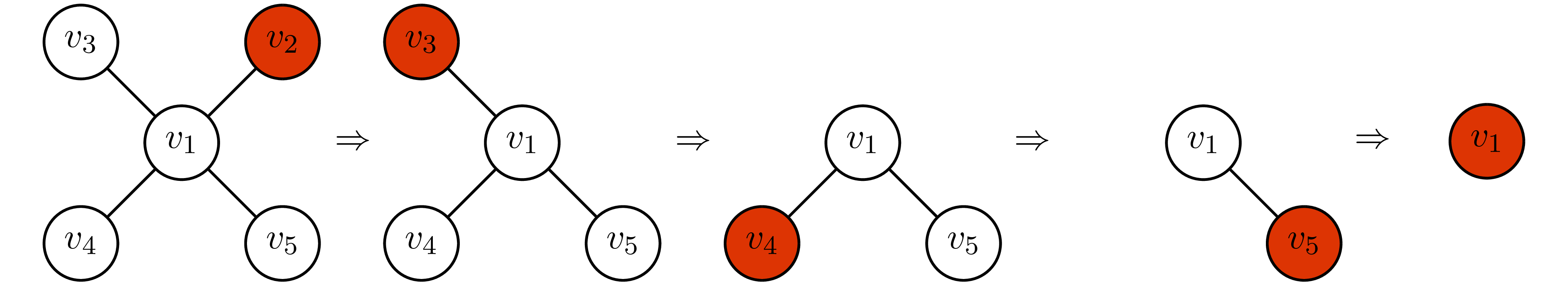In case 1, we add 6 edges during the process of elimination. However, in case 2, we do not have to add any edges. We call the elimination order that does not introduce new edges during the vertex elimination the perfect elimination ordering.

An elimination order is call the perfect elimination ordering if there is no edge being added during the process of vertex elimination.

he idea of vertex elimination is tightly related to Cholesky factorization. In fact, if the graphical structure of ${\bf A}$ has the perfect elimination ordering, the Cholesky factor of ${\bf A}$ will have the same structure if rows and columns of ${\bf A}$ are arranged in perfect elimination order. This idea is illustrated as follow.

Using the same graph and elimination order in Example 1, and let the number in each vertex denotes the order of elimination.

Case 1: rows and columns of ${\bf A}$ are not in perfect elimination ordering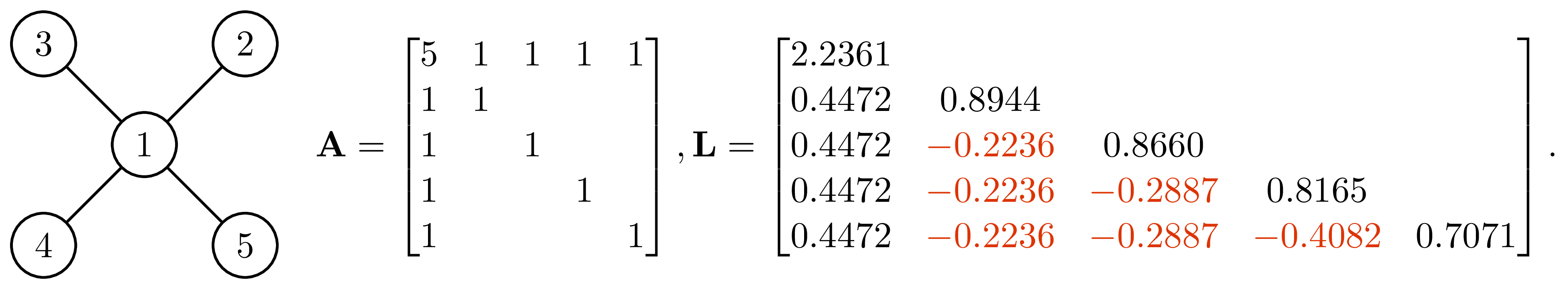Case 2: rows and columns of ${\bf A}$ are in perfect elimination ordering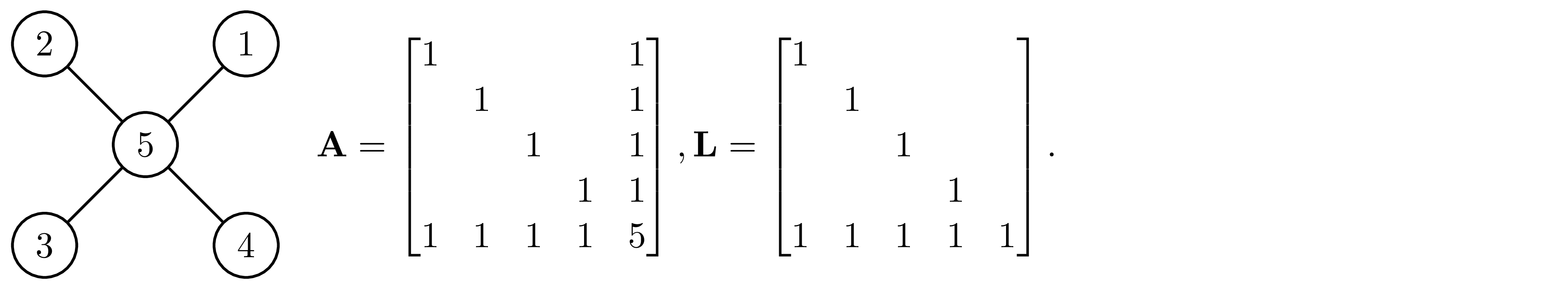The element in matrix that were zeros in ${\bf A}$ but become non-zero in ${\bf L}$ is called fill-in (the elements marked in red in case 1). In case 1, the Cholesky factor has 6 fill-in and become fully dense. However, if we permute the ${\bf A}$ into the perfect elimination order as shown in case 2, the Cholesky factor has no fill-in. Here, we give a conceptual explanation for this result, for more detailed and rigorous proof, see Appendix A. Partition ${\bf A}$ and compute its Schur complement, we haveThis expression gives us the first column of Cholesky factor $\sqrt{d_1}\begin{bmatrix}1\\\frac{1}{d_1}{\bf b}_1\end{bmatrix}$. Obviously, the fill-in are cause by the term ${\bf b}_1{\bf b}_1^\mathrm{T}$ in ${\bf D}_1$. Let ${\bf a}_1=\begin{bmatrix}d_1\\{\bf b}_1\end{bmatrix}$ be the first column of ${\bf A}$. Because of the term ${\bf b}_1{\bf b}_1^\mathrm{T}$ in ${\bf D}_1$, if $(1,i)$-th and $(1,j)$-th elements in ${\bf a}_1$ is not zero, $i,j>1$, then the $(i,j)$-th element of ${\bf D}_1$ becomes nonzero. Graphically, it is equivalent to saying that if $(1,i)$, $(1,j)$ are connected, then $(i,j)$ will become connected after eliminating vertex 1, which is exactly the process of vertex elimination. The Cholesky factor can be obtained by performing the similar partition on ${\bf D}_1$ then compute its Schur complement to obtain ${\bf D}_2$, and then repeat this process until we get ${\bf D}_n$ (see Appendix A for details). If rows and columns of ${\bf A}$ are in perfect elimination order, then each ${\bf D}_1,\ldots,{\bf D}_n$ will have no fill-in, it follows that the Cholesky factor of ${\bf A}$ will have no fill-in as well.

Let $\boldsymbol{A}\succ 0$ be a symmetric matrix. If $\boldsymbol{A}$ is in perfect elimination ordering, then its Cholesky factor $\boldsymbol{L}$ has no fill-in.

In the following, we use the matrix ${\bf A}$ in the case 1 above to illustrate this idea. Partition ${\bf A}$ and compute its Schur complement, we have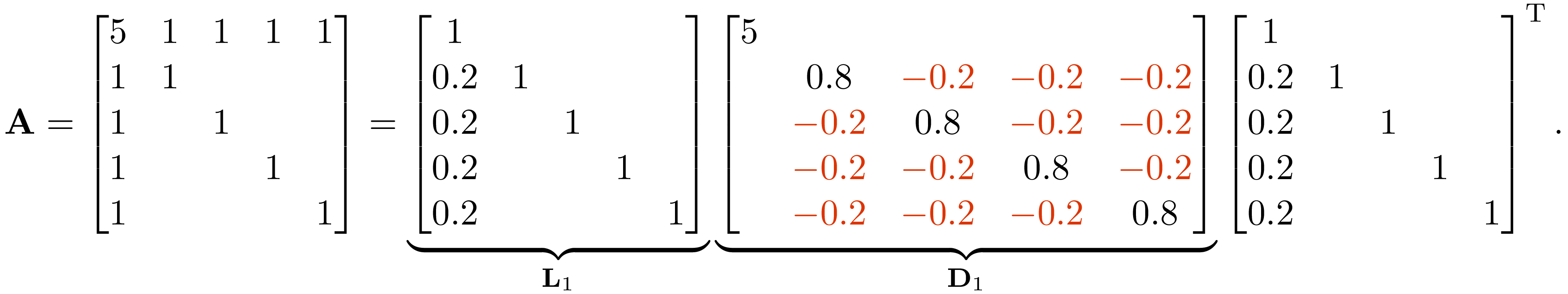Observe that the graphical structure of ${\bf C}_1-\frac{1}{d_1}{\bf b}_1{\bf b}_1^\mathrm{T}$ is the graphical structure of ${\bf A}$ after eliminating vertex $1$.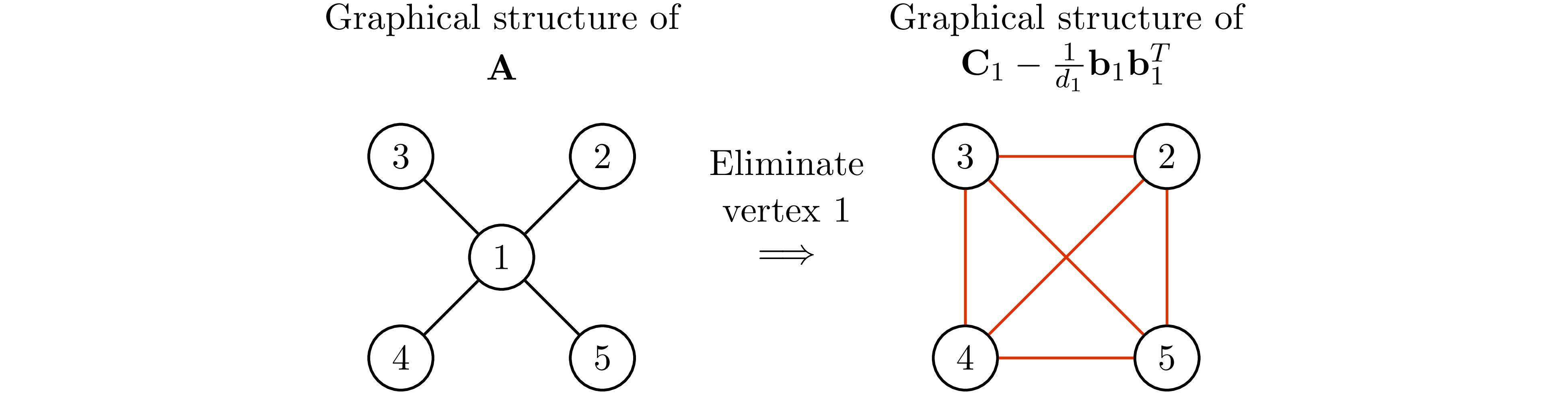Notice that not every graph has perfect elimination ordering, and the perfect elimination ordering (if exists) is not unique in general. Fulkerson et at. 2 showed that graphs that have the perfect elimination ordering are exactly the chordal graphs.

An undirected graph is chordal if and only if it has the perfect elimination ordering.

Any chordal graph $\mathrm{G(V,E)}$ can be turn into a tree that has the clique (maximal complete subgraph) of $\mathrm G$ being its vertex. This idea is illustrated in the following example borrowed from 3.

The chordal graph in p.29 of 3 and its clique tree. Let $W$ denotes a set of cliques.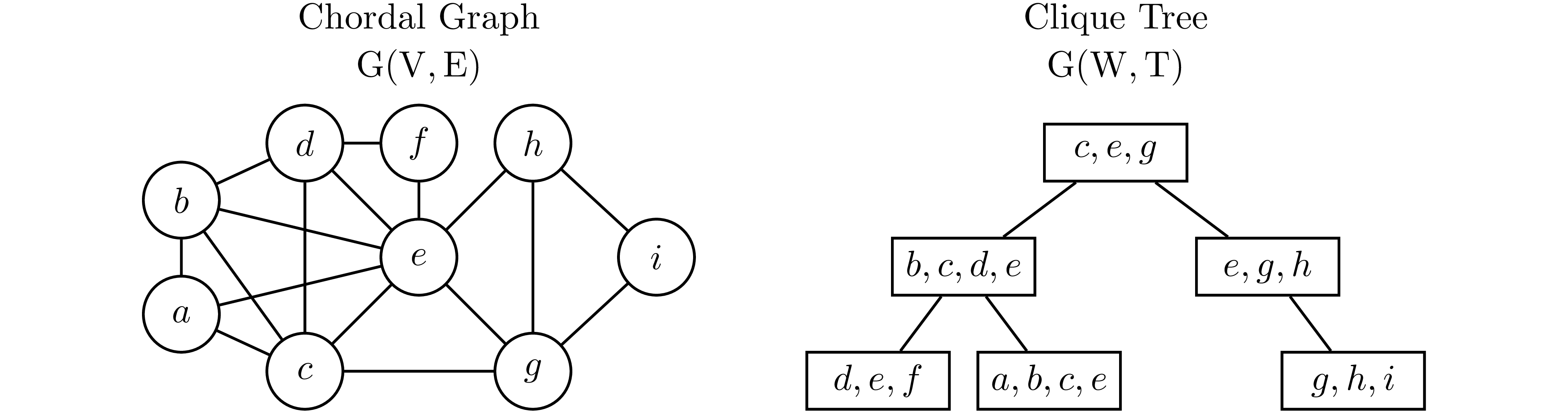In Example 3, observe that for any $v\in\mathrm V$, every vertex in the clique tree contains $v$ induce a subtree. Therefore, if $v$ is contained in two cliques $W_1$ and $W_2$, then $v$ has to be contained in every cliques that are in the path from $W_1$ to $W_2$ on the clique tree. This is known as induce subtree or running intersection property.

The induce subtree of vertex $e$ and $c$.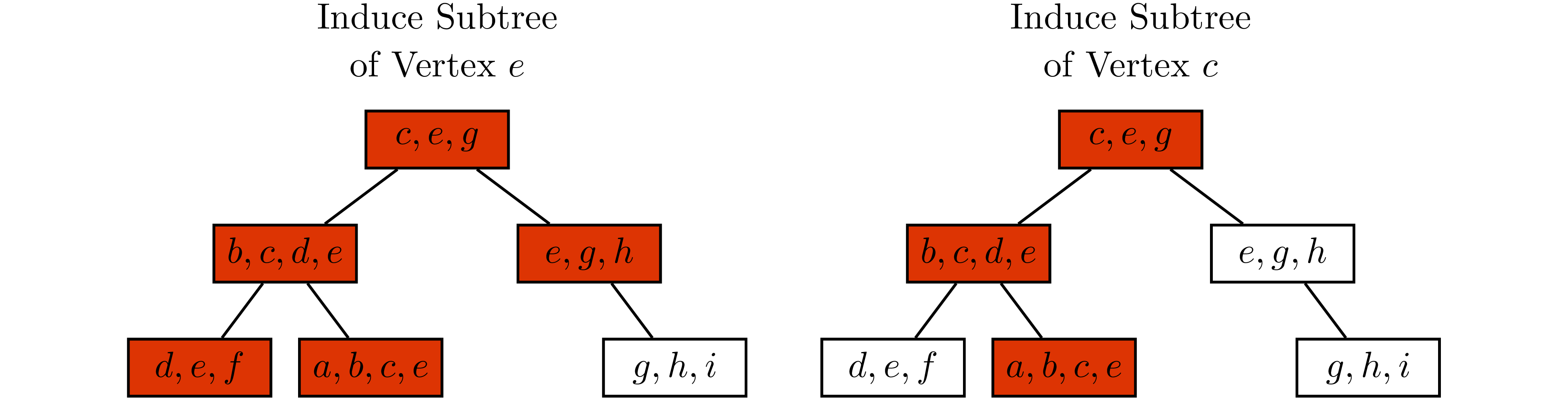Suppose ${\bf C}, {\bf A}_1,\ldots,{\bf A}_m\in \mathrm{S_E^n}$. 4 5 6 7 proposed to reformulated SDP aswhere $\mathrm{P_E(S_+^n)}=\left\{\mathrm{P_E}({\bf X}) \mid {\bf X} \succeq 0\right\}$ is the cone of matrices in $\mathrm{S_E^n}$ that have a positive semidefinite completion.

A projection ${\bf Y}$ of a matrix ${\bf X}$ onto a sparsity pattern is denoted ${\bf Y}=\mathrm{P_E({\bf X})}$, i.e., $Y_{i,j}=X_{i,j}$ if $(i,j)\in\mathrm E$ and otherwise $Y_{i,j}=0$.

If we further suppose $\mathrm{G(V,E)}$ is chordal. Let $W_1,\ldots,W_l$ denotes the cliques of $\mathrm G$, and ${\bf X}[W_j,W_j]$ denotes sub-matrices corresponding to clique $W_j$. Grone et al. 7 showed that this problem can be further relaxed into the optimization problem that only enforce the positive semidefiniteness constraint on each ${\bf X}[W_j,W_j]$$\boldsymbol{X}$ is positive semidefinite completable, or $\boldsymbol{X}\in P_E(S_+^n)$, if and only if $\boldsymbol{X}[W_j,W_j]\succeq 0$ for all $j=1,\ldots,l$.

By Theorem 2, the relaxation above is always possible, then it follows that the relaxation is tight because ${\bf C}\bullet{\bf X}={\bf C}\bullet \mathrm{P_E({\bf X})}$. For simplicity, let ${\bf X}_j={\bf X}[W_j,W_j]$. For some ${\bf C}_j,{\bf A}_{ij}\in\mathbb R^{|W_j|\times |W_j|}$, we can further rewrite this problem into the optimization problem over ${\bf X}_j$'s.where $\mathcal N_{ij}(X_i)$ denotes the part of ${\bf X}_i$ overlaps with ${\bf X}_j$. The reformulation above is called the clique tree conversion (CTC) for SDP. It is worth noting that we only have to impose the overlaping constraint $\mathcal N_{ij}(X_i)=\mathcal N_{ji}(X_j)$ along the edge of clique tree because of the induce subtree properties. Example 5 gives a concrete example for this reformulation.

Let the graph $\mathrm{G(V,E)}$ shown in the left-hand side be the graphical structure of ${\bf C},{\bf A}_1,\ldots,{\bf A}_m$. Notice that $\mathrm G$ is chordal and has two cliques $W_1$ and $W_2$. The corresponding ${\bf X}_1={\bf X}[W_1,W_1]$, ${\bf X}_2={\bf X}[W_2,W_2]$ and $\mathcal N_{1,2}({\bf X}_1)=\mathcal N_{2,1}({\bf X}_2)$ are shown in the right-hand side.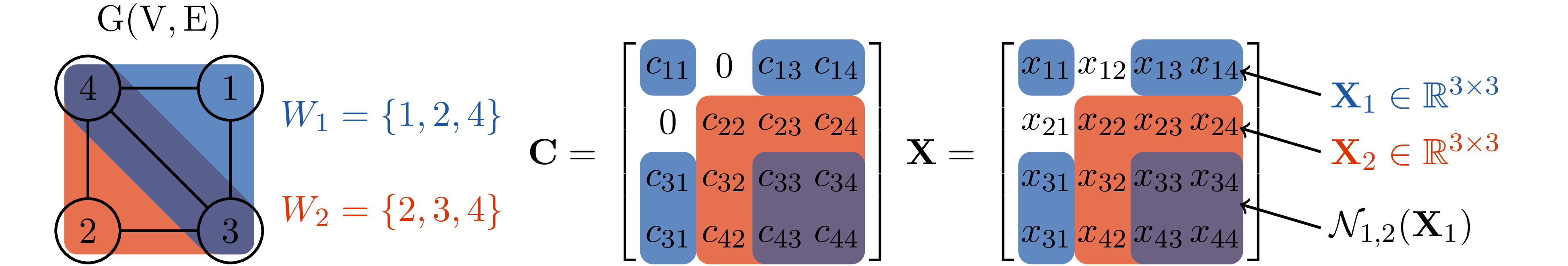Notice that the ${\bf X}$ obtained from 2 does not guarantee to be positive semidefinite; therefore, when ${\bf X}$ is not positive semidefinite, we need to project ${\bf X}$ back to the positive semidefinite cone. This process is called positive semidefinite completion and is given as followFigure 1 illustrate the process of solving SDP through the clique tree conversion 2 following by the positive semidefinite completion 3The graphical illustration of solving SDP 1 through clique tree conversion 2 and positive semidefinite completion 3. The feasible set of 1 and 2 are shown in blue and orange respectively. $\widehat {\bf X}$ denotes an optimizer of 2, and $\widehat {\bf Z}$ denotes the solution obtained after 3, which is an optimizer of 1. Obviously, if $\widehat{\bf X}$ were in blue set, then we can skip 3.

The clique tree conversion can be vectorizes into the standard form of linear conic programwhere $\mathcal K=\mathrm{S^{|W_1|}_+}\times,\ldots,\times, \mathrm{S_+}^{|\mathrm W_l|}$. Let $f(n)=n(n+1)/2$ be a function outputs the number of elements in the lower triangular part of $n\times n$ matrix. $\text{svec}({\bf A})\in\mathbb R^{f(n)}$ be the weighted vectorization of the lower triangular part of $n\times n$ matrix ${\bf A}$ such that $\text{svec}({\bf A})^\mathrm{T}\text{svec}({\bf B})={\bf A}\bullet{\bf B}$. Then ${\bf c},{\bf x}\in\mathbb R^{n'}$, ${\bf A}\in\mathbb R^{m\times n'}$, $n'=\sum_{j=1}^lf(|W_j|)$ is given byIn addition ${\bf N}\in\mathbb R^{m'\times n'}$ is a block matrix, where $m'=\sum_{i=1}^lf(|W_i\cap W_{p(i)}|)$ and $p(i)$ is the parent of vertex $i$ in the clique tree. ${\bf N}$ and its blocks ${\bf N}_{i,j}\in\mathbb R^{f(|W_i\cap W_{p(i)}|)\times f(|W_j|)}$ are defined asThe constraint $\sum_{j=1}^l{\bf A}_{ij}\bullet{\bf X}_j=b_i$ is said to be partially separable if and only if there exists some ${\bf A}_i'$ and $i'\in\{1,\ldots,l\}$ such thatFrieze et at. 8 proposed a randomized algorithm to approximate MAX k-CUP base on solvingObserve that each constraint affect a single matrix element, therefore this problem is partially separable.

When SDP is partially separable, the blocks of matrix ${\bf A}$ in 4 becomesZhang et al. 1 proposed to exploit sparsity structure of ${\bf H}$ using the dualizing technique of Lofberg 9. The dual of 4 is given bywhere $\mathcal K^*=\mathcal K$ is the dual cone of $\mathcal K$. The dualization technique of Lofberg 9 swap the role played by primal and dual problem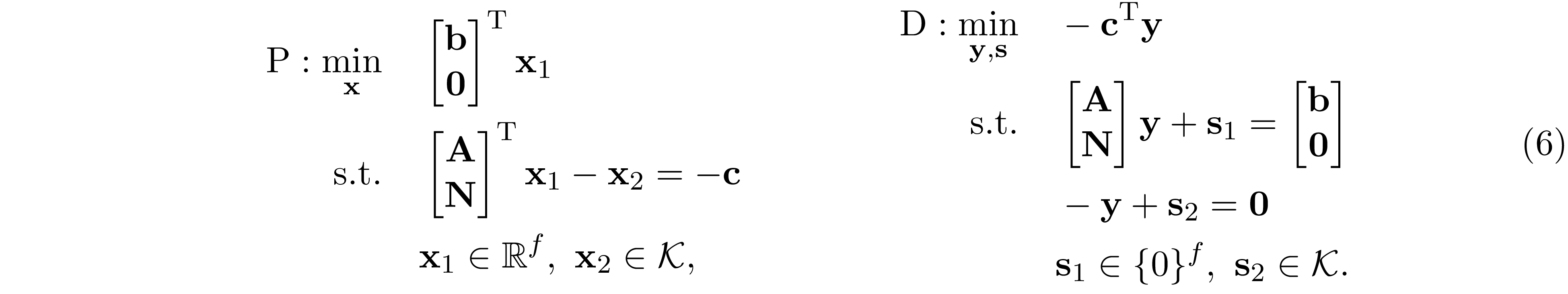where $f=m+m'$ is the number of equality constraint. Observe that the dual problem of 6 is exactly the same as the primal problem in 5. Applying the IPM to solve 6. The normal equation we need to solve at each iteration takes the formwhere ${\bf D}_s$ is a block diagonal matrix such that ${\bf D}_s=\text{diag}({\bf D}_{s1},\ldots,{\bf D}_{sl})$, ${\bf D}_{si}\in\mathbb R^{f(|W_i|)\times f(|W_i|)}$ and ${\bf D}_{s,i}\succeq 0$. ${\bf D}_f$ is low-rank perturbation such that ${\bf D}_f=\sigma{\bf I}+{\bf w}{\bf w}^\mathrm{T}$. Letand apply Sherman-Morrison-Woodbury, we haveThe block sparsity pattern of ${\bf N}^\mathrm{T}{\bf N}$ coincides with the tree graph $\mathrm{G(W,T)}$, and ${\bf A}^\mathrm{T}{\bf A}$ is a block diagonal matrix under the partially separable assumption. As a result, the block sparsity pattern of ${\bf H}$ is chordal. Example 1 gives a concrete example.

Using the chordal graph $\mathrm{G(V,E)}$ and its tree graph $\mathrm{G(W,T)}$ in Example 3 $(n=9,l=6)$. Suppose $m=5$ and $\{i'\}=\{2,4,1,2,5\}$, then the ${\bf A}$ and ${\bf A}^\mathrm{T}{\bf A}$ has the following structure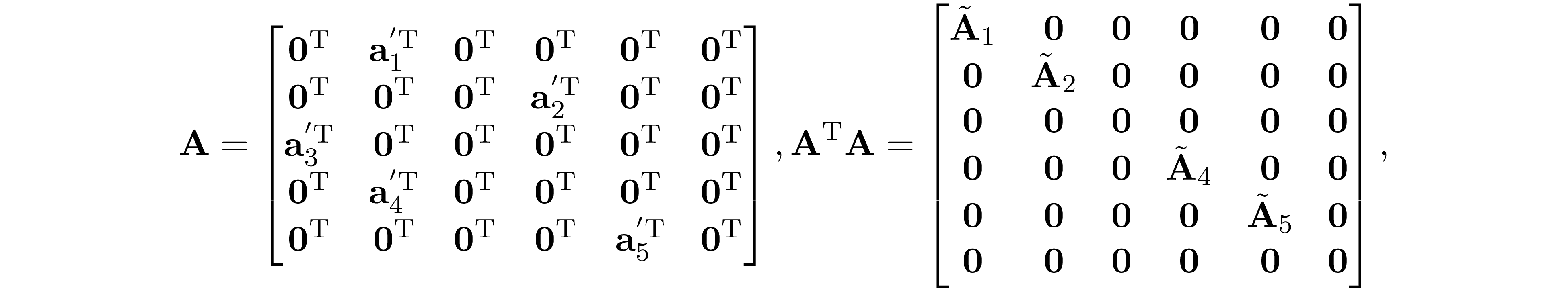where ${\bf a}_i'=\text{svec}({\bf A}'_i)$, and $\tilde{\bf A}_i\in\mathbb R^{f(|W_i|)\times f(|W_i|)}$ are some dense matrix. Suppose we index the tree graph $\mathrm{G(W,T)}$ in post-order, then ${\bf N}$ and ${\bf N}^\mathrm{T}{\bf N}$ has the following structure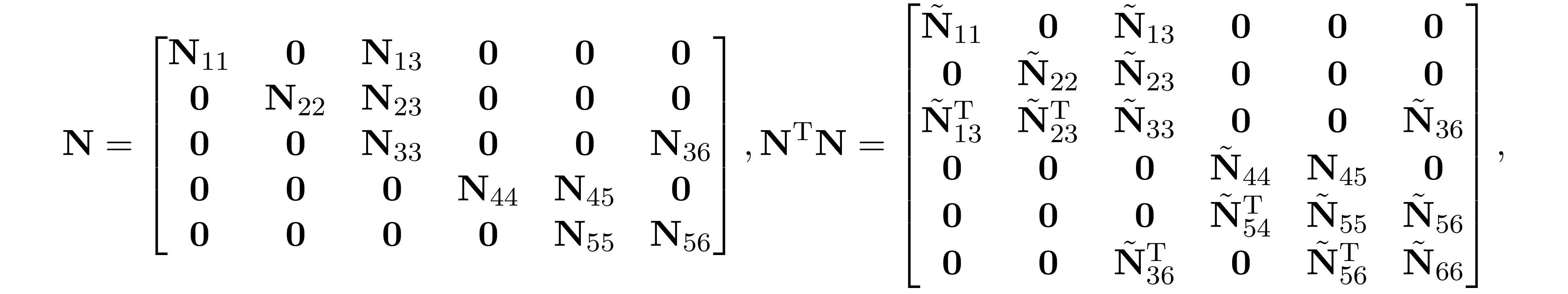where $\tilde{\bf N}_{i,j}\in\mathbb R^{f(|W_i|)\times f(|W_j|)}$ are some dense matrix. Therefore, ${\bf D}_s+{\bf A}^\mathrm{T}{\bf A}+{\bf N}^\mathrm{T}{\bf N}$ has the following block structure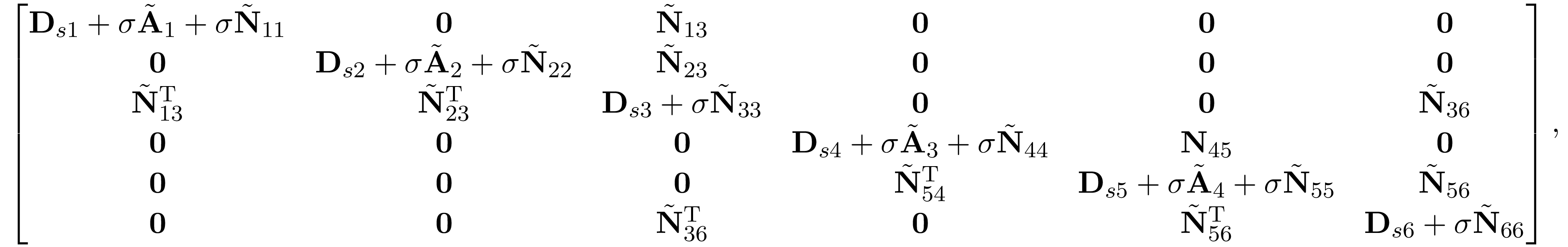coincides with the graphical structure of tree graph $\mathrm{G(W,T)}$.
Let $\boldsymbol{H}\succ 0$ be a symmetric block matrix. If the block sparsity pattern of $\boldsymbol{H}$ is chordal, then its Cholseky factor has no block fill.
Adapt the proof in Appendix A. Partition ${\bf H}$ and compute its Schur complement, we haveThe block fill-in is caused by ${\bf B}_1{\bf D}_{11}^{-1}{\bf B}_1^\mathrm{T}$ in ${\bf D}_1$. Then it follows that when the block sparsity pattern of ${\bf H}$ is chordal, then the block sparsity pattern of ${\bf C}_1$ will be the same as the block sparsity pattern of ${\bf B}_1{\bf D}_{11}^{-1}{\bf B}_1^\mathrm{T}$. The Cholesky factor can be obtained by applying the similar partition on ${\bf D}_1$ and computes its Schur complement, and then repeat this process until we obtain the block diagonal matrix ${\bf D}_n$.
$\square$

Observe that evaluating ${\bf H}^{-1}{\bf q}$ is the same as solving ${\bf q}'$ such that ${\bf H}{\bf q}'={\bf q}$. ${\bf q}'$ can be solved by first factorizing ${\bf H}={\bf L}{\bf L}^\mathrm{T}$, and then solve ${\bf L}{\bf b}={\bf q}$ following by ${\bf L}^\mathrm{T}{\bf q}'={\bf b}$. By Lemma 2, ${\bf L}$ has no block fill; therefore, ${\bf L}$ can be factorized in $O(\beta^3n)$ and ${\bf q}'$ can be solved in $O(\beta^2n)$, where $\beta=\max_jf(|W_j|)$. This result allows us to evaluate $\Delta{\bf y}$ in 7 in $O(\omega^6n)$ time, where $\omega=\max_j|W_j|$ and $\beta\leq\omega^2$. As a result, the computational complexity to obtain the $\epsilon$-accuracy solution using IPM is $O(w^6n^{1.5}\log(1/\epsilon))$. Notice that Zhang's method 1 is better than Andersen's method 10 when the number of equality constraints $m$ is large. Specifically, when $m$ is of $O(\omega n)$ then Andersen's method requires $O(\omega^3n^{3.5}\log(1/\epsilon))$ time complexity, which is comparable to the cubic time complexity of direct IPM when the number of constraint $m$ is of $O(n)$.

Suppose ${\bf A}\in\mathrm{S_{E}^n}$. Partition ${\bf A}$ and computes its Schur complement, we haveWe can easily see that ${\bf L}_1+{\bf L}_1^\mathrm T\in\mathrm{S_{E}^n}$. Let $\mathrm{E_1}$ be the sparsity pattern of ${\bf D}_1$, then $\mathrm{E_1}$ is simply the union of sparsity pattern $\mathrm{E}$ and any new edges introduced by the outer product of ${\bf b}_1$Because ${\bf A}$ is in perfect elimination ordering we have $\{(i,j)\mid (1,i)\in\mathrm E,\ (1,j)\in\mathrm E\}\subseteq\mathrm E$, therefore $\mathrm E_1=\mathrm E$ and ${\bf D}_1\in\mathrm{S_{E}^n}$. Similarly, we can apply the same partition on ${\bf D}_1$ and factor it as ${\bf D}_1=\widetilde{\bf L}_2{\bf D}_2\widetilde{\bf L}^\mathrm{T}_2$ where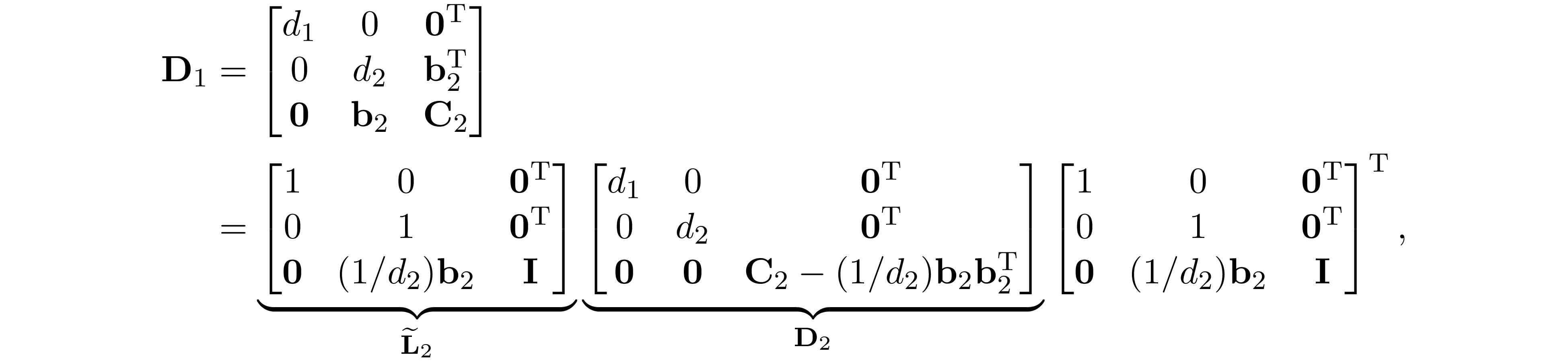and let ${\bf L}_2={\bf L}_1\widetilde{\bf L}_2$, we have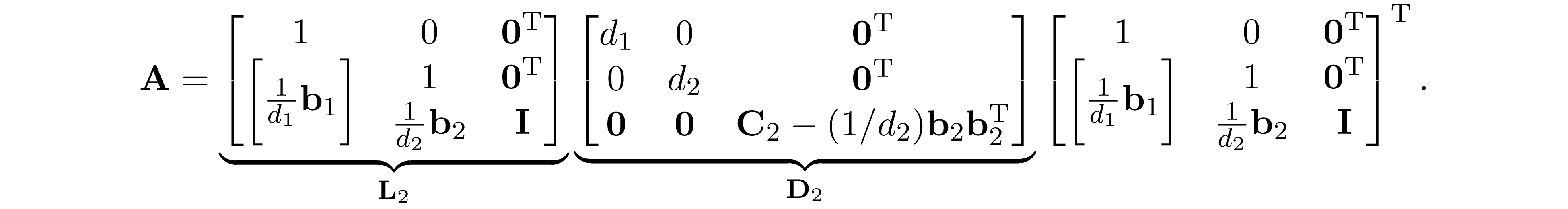Let $\mathrm{E_2}$ be the sparsity pattern of ${\bf D}_2$, becasue ${\bf A}$ is in perfect elimination ordering we haveRepeating this procedure $n$ times, we have ${\bf A}={\bf L}_n{\bf D}_n{\bf L}_n^\mathrm T$, where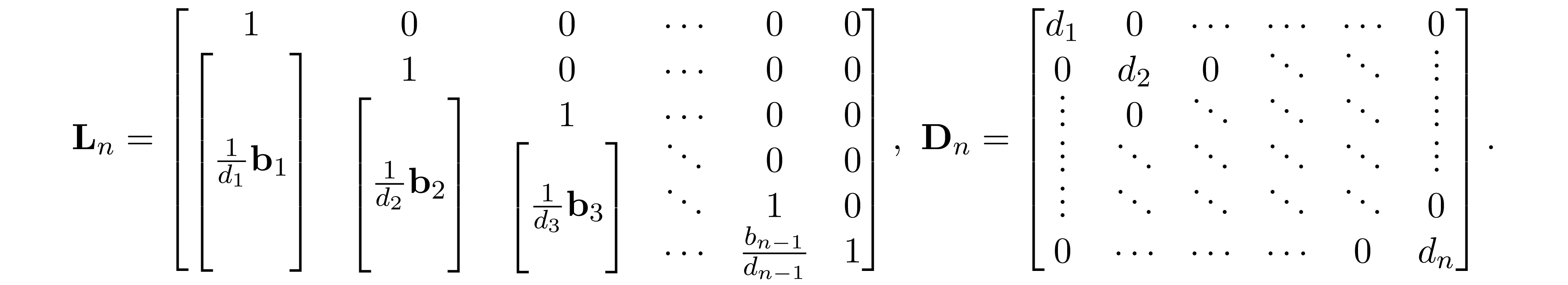Because ${\bf A}$ is in perfect elimination ordering we have $\mathrm E_n =\mathrm E_{n-1} = \ldots = \mathrm E_1= \mathrm E$. Since ${\bf D}_n$ is diagonal and ${\bf L}={\bf L}_n{\bf D}_n^{\frac{1}{2}}$, we conclude that ${\bf L}+{\bf L}^\mathrm T\in\mathrm{S^n_E}$; therefore, the Cholesky factor ${\bf L}$ has no fill-in.
$\square$
1 Zhang, R.Y., Lavaei, J. "Sparse semidefinite programs with guaranteed near-linear time complexity via dualized clique tree conversion." Mathematical Programming 188, 351-393 (2021).
2 D. R. Fulkerson and O. Gross. "Incidence matrices and interval graphs." Pacific Journal of Mathematics, 15(3):835-855, 1965.
3 Lieven Vandenberghe and Martin S. Andersen, "Chordal Graphs and Semideﬁnite Optimization," 2015.
4 Samuel Burer, "Semideﬁnite programming in the space of partial positive semideﬁnite matrices," SIAM J. Optim., vol. 14, pp. 139-172, 2003.
5 Mituhiro Fukuda, Masakazu Kojima, Kazuo Murota, and Kazuhide Nakata, "Exploiting sparsity in semideﬁnite programming viamatrix completion i: General framework," vol. 11, no. 3, 2000.
6 Mituhiro Fukuda, Masakazu Kojima, Kazuhide Nakata, Katsuki Fujisawa and Kazuo Murota, "Exploiting sparsity in semideﬁnite programming viamatrix completion ii: implementation and numerical results," 2003.
7 Gun Srijuntongsiri and Stephen A. Vavasis, "A fully sparse implementation of a primal-dual interior-point potential reduction method for semideﬁnite programming," ArXiv, vol. abs/cs/0412009, 2004.
8 Alan Frieze and M. Jerrum, "Jerrum, m.: Improved approximation algorithms for max k-cut and max bisection. algorithmica 18(1), 67-81," Algorithmica, vol. 18, pp. 67-81, 05 1997.
9 Johan Lofberg, "Dualize it: software for automatic primal and dual conversions of conic programs," OptimizationMethods andSoftware, vol. 24, no. 3, pp. 313-325, 2009.
10 Martin Andersen, Joachim Dahl, and Lieven Vandenberghe, "Implementation of nonsym- metric interior-point methods for linear optimization over sparse matrix cones, Mathematical Programming Computation, vol. 2, pp. 167-201, 12 2010.